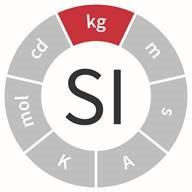An official website of the United States governmentThe .gov means it’s official.
Federal government websites often end in .gov or .mil. Before sharing sensitive information, make sure you’re on a federal government site.The site is secure.
The https:// ensures that you are connecting to the official website and that any information you provide is encrypted and transmitted securely.

# SI Units - Mass

## ShareThe kilogram (kg) is defined by taking the fixed numerical value of the Planck constant h to be 6.62607015 ×10−34 when expressed in the unit J s, which is equal to kg m2 s−1, where the meter and the second are defined in terms of c and ∆νCs.

The primary standard of mass for this country is United States Prototype Kilogram 20, which is a platinum-iridium cylinder kept at NIST. The kilogram, originally defined as the mass of one cubic decimeter of water at the temperature of maximum density, was known as the Kilogram of the Archives. It was replaced after the International Metric Convention in 1875 by the International Prototype Kilogram which became the unit of mass without reference to the mass of a cubic decimeter of water or to the Kilogram of the Archives. Each country that subscribed to the International Metric Convention was assigned one or more copies of the international standards; these are known as National Prototype Meters and Kilograms. Learn more about the history and current definition of the kilogram.

Among the SI base units, the kilogram (kg) is the only one whose name and symbol, for historical reasons, include a prefix. "Kilo" the SI prefix for 1000 or 103. Names and symbols for decimal multiples and submultiples of the unit of mass are formed by attaching prefix names to the unit name "gram," and prefix symbols to the unit symbol "g." Learn more about this historical quirk.

Units of Mass
10 milligrams (mg) = 1 centigrams (cg)
10 centigrams = 1 decigrams (dg) = 100 milligrams
10 decigrams = 1 gram (g)
10 decigrams = 1000 milligrams
10 grams = 1 dekagrams (dag)
10 dekagrams = 1 hectogram (hg)
10 dekagrams = 100 grams
10 hectograms = 1 kilogram (kg)
10 hectograms = 1000 grams
1000 kilograms = 1 megagram (Mg) or 1 metric ton (t)Physicist Richard Steiner adjusts the electronic kilogram, an experimental apparatus for defining mass in terms of the basic properties of nature.

## For Students and Teachers

### League of SI Superheroes - Monsieur Kilogram

This comic book-style video animation series has been developed to help middle school students learn about the 7 SI base measurement units. With his immensely strong arms, Monsieur Kilogram is the master of mass. The kilogram is a cylinder of special metal about 39 millimeters wide by 39 millimeters tall that serves as the world's mass standard.FAQ - What is the difference between the terms "mass" and "weight"?
The mass of a body is a measure of its inertial property or how much matter it contains. The weight of a body is a measure of the force exerted on it by gravity or the force needed to support it. Gravity on earth gives a body a downward acceleration of about 9.8 m/s2. In common parlance, weight is often used as a synonym for mass in weights and measures. For instance, the verb “to weigh” means “to determine the mass of” or “to have a mass of.” The incorrect use of weight in place of mass should be phased out, and the term mass used when mass is meant. The SI unit of mass is the kilogram (kg). In science and technology, the weight of a body in a particular reference frame is defined as the force that gives the body an acceleration equal to the local acceleration of free fall in that reference frame. Thus, the SI unit of the quantity weight defined in this way (force) is the newton (N).

## Contacts

• Metric Program
Created March 30, 2011, Updated December 9, 2019